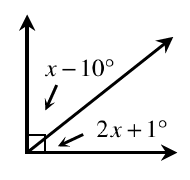### Home > GC > Chapter 1 > Lesson 1.3.3 > Problem1-124

1-124.Rosalinda examined the angles at right and wrote the equation below.

$(2x+1º)+(x-10º)=90º$

1. Does her equation make sense? If so, explain why her equation must be true. If it is not correct, determine what is incorrect and write the equation.

Is a right angle (as illustrated above), equal to $90º$?

Yes. This means Rosalinda's equation makes sense, because the two angles make up a $90º$ angle.

2. If you have not already done so, solve her equation, clearly showing all your steps. What are the measures of the two angles?

Simplify without the parentheses and combine like terms.  Then solve for $x$. One of the angles is $x-10$. The other is $2x+1$. The sum of the two angles is $90$.

After solving for $x$, substitute your answer into the original equation to verify it is correct.